» Developer guides / KerasCV / CutMix, MixUp, and RandAugment image augmentation with KerasCV

# CutMix, MixUp, and RandAugment image augmentation with KerasCV

Author: lukewood
Date created: 2022/04/08
Description: Use KerasCV to augment images with CutMix, MixUp, RandAugment, and more.View in ColabGitHub source

## Overview

KerasCV makes it easy to assemble state-of-the-art, industry-grade data augmentation pipelines for image classification and object detection tasks. KerasCV offers a wide suite of preprocessing layers implementing common data augmentation techniques.

Perhaps three of the most useful layers are `keras_cv.layers.CutMix`, `keras_cv.layers.MixUp`, and `keras_cv.layers.RandAugment`. These layers are used in nearly all state-of-the-art image classification pipelines.

This guide will show you how to compose these layers into your own data augmentation pipeline for image classification tasks. This guide will also walk you through the process of customizing a KerasCV data augmentation pipeline.

## Imports & setup

This tutorial requires you to have KerasCV installed:

``````pip install keras-cv
``````

We begin by importing all required packages:

``````import keras_cv
import matplotlib.pyplot as plt
import tensorflow as tf
import tensorflow_datasets as tfds
from tensorflow import keras
from tensorflow.keras import applications
from tensorflow.keras import losses
from tensorflow.keras import optimizers
``````

This guide uses the 102 Category Flower Dataset for demonstration purposes.

To get started, we first load the dataset:

``````BATCH_SIZE = 32
AUTOTUNE = tf.data.AUTOTUNE
tfds.disable_progress_bar()
data, dataset_info = tfds.load("oxford_flowers102", with_info=True, as_supervised=True)
train_steps_per_epoch = dataset_info.splits["train"].num_examples // BATCH_SIZE
val_steps_per_epoch = dataset_info.splits["test"].num_examples // BATCH_SIZE
``````

Next, we resize the images to a constant size, `(224, 224)`, and one-hot encode the labels. Please note that `keras_cv.layers.CutMix` and `keras_cv.layers.MixUp` expect targets to be one-hot encoded. This is because they modify the values of the targets in a way that is not possible with a sparse label representation.

``````IMAGE_SIZE = (224, 224)
num_classes = dataset_info.features["label"].num_classes

def to_dict(image, label):
image = tf.image.resize(image, IMAGE_SIZE)
image = tf.cast(image, tf.float32)
label = tf.one_hot(label, num_classes)
return {"images": image, "labels": label}

def prepare_dataset(dataset, split):
if split == "train":
return (
dataset.shuffle(10 * BATCH_SIZE)
.map(to_dict, num_parallel_calls=AUTOTUNE)
.batch(BATCH_SIZE)
)
if split == "test":
return dataset.map(to_dict, num_parallel_calls=AUTOTUNE).batch(BATCH_SIZE)

dataset = data[split]
return prepare_dataset(dataset, split)

``````

Let's inspect some samples from our dataset:

``````def visualize_dataset(dataset, title):
plt.figure(figsize=(6, 6)).suptitle(title, fontsize=18)
for i, samples in enumerate(iter(dataset.take(9))):
images = samples["images"]
plt.subplot(3, 3, i + 1)
plt.imshow(images.numpy().astype("uint8"))
plt.axis("off")
plt.show()

visualize_dataset(train_dataset, title="Before Augmentation")
``````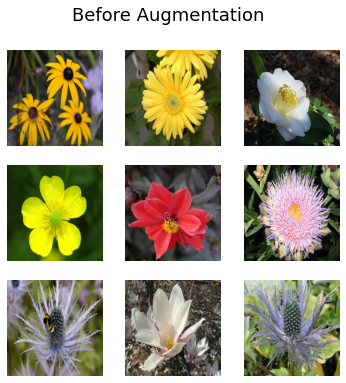Great! Now we can move onto the augmentation step.

## RandAugment

RandAugment has been shown to provide improved image classification results across numerous datasets. It performs a standard set of augmentations on an image.

To use RandAugment in KerasCV, you need to provide a few values:

• `value_range` describes the range of values covered in your images
• `magnitude` is a value between 0 and 1, describing the strength of the perturbations applied
• `augmentations_per_image` is an integer telling the layer how many augmentations to apply to each individual image
• (Optional) `magnitude_stddev` allows `magnitude` to be randomly sampled from a distribution with a standard deviation of `magnitude_stddev`
• (Optional) `rate` indicates the probability to apply the augmentation applied at each layer.

You can read more about these parameters in the `RandAugment` API documentation.

Let's use KerasCV's RandAugment implementation.

``````rand_augment = keras_cv.layers.RandAugment(
value_range=(0, 255),
augmentations_per_image=3,
magnitude=0.3,
magnitude_stddev=0.2,
rate=0.5,
)

def apply_rand_augment(inputs):
inputs["images"] = rand_augment(inputs["images"])
return inputs

``````

Finally, let's inspect some of the results:

``````visualize_dataset(train_dataset, title="After RandAugment")
``````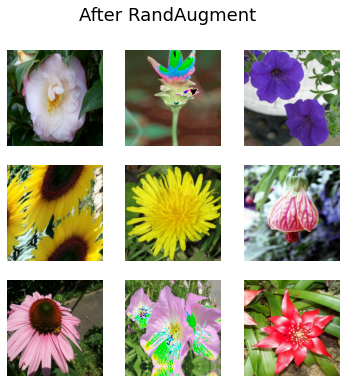Try tweaking the magnitude settings to see a wider variety of results.

## CutMix and MixUp: generate high-quality inter-class examples

`CutMix` and `MixUp` allow us to produce inter-class examples. `CutMix` randomly cuts out portions of one image and places them over another, and `MixUp` interpolates the pixel values between two images. Both of these prevent the model from overfitting the training distribution and improve the likelihood that the model can generalize to out of distribution examples. Additionally, `CutMix` prevents your model from over-relying on any particular feature to perform its classifications. You can read more about these techniques in their respective papers:

In this example, we will use `CutMix` and `MixUp` independently in a manually created preprocessing pipeline. In most state of the art pipelines images are randomly augmented by either `CutMix`, `MixUp`, or neither. The function below implements both.

``````cut_mix = keras_cv.layers.CutMix()
mix_up = keras_cv.layers.MixUp()

def cut_mix_and_mix_up(samples):
samples = cut_mix(samples, training=True)
samples = mix_up(samples, training=True)
return samples

visualize_dataset(train_dataset, title="After CutMix and MixUp")
``````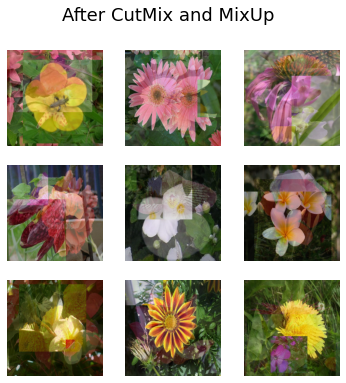Great! Looks like we have successfully added `CutMix` and `MixUp` to our preprocessing pipeline.

Perhaps you want to exclude an augmentation from `RandAugment`, or perhaps you want to include the `keras_cv.layers.GridMask` as an option alongside the default `RandAugment` augmentations.

KerasCV allows you to construct production grade custom data augmentation pipelines using the `keras_cv.layers.RandomAugmentationPipeline` layer. This class operates similarly to `RandAugment`; selecting a random layer to apply to each image `augmentations_per_image` times. `RandAugment` can be thought of as a specific case of `RandomAugmentationPipeline`. In fact, our `RandAugment` implementation inherits from `RandomAugmentationPipeline` internally.

In this example, we will create a custom `RandomAugmentationPipeline` by removing `RandomRotation` layers from the standard `RandAugment` policy, and substitute a `GridMask` layer in its place.

As a first step, let's use the helper method `RandAugment.get_standard_policy()` to create a base pipeline.

``````layers = keras_cv.layers.RandAugment.get_standard_policy(
value_range=(0, 255), magnitude=0.75, magnitude_stddev=0.3
)
``````

First, let's filter out `RandomRotation` layers

``````layers = [
layer for layer in layers if not isinstance(layer, keras_cv.layers.RandomRotation)
]
``````

Next, let's add `keras_cv.layers.GridMask` to our layers:

``````layers = layers + [keras_cv.layers.GridMask()]
``````

Finally, we can put together our pipeline

``````pipeline = keras_cv.layers.RandomAugmentationPipeline(
layers=layers, augmentations_per_image=3
)

def apply_pipeline(inputs):
inputs["images"] = pipeline(inputs["images"])
return inputs
``````

Let's check out the results!

``````train_dataset = load_dataset().map(apply_pipeline, num_parallel_calls=AUTOTUNE)
visualize_dataset(train_dataset, title="After custom pipeline")
``````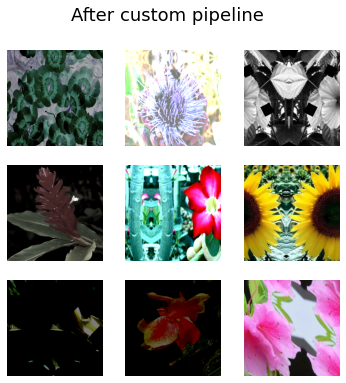Awesome! As you can see, no images were randomly rotated. You can customize the pipeline however you like:

``````pipeline = keras_cv.layers.RandomAugmentationPipeline(
augmentations_per_image=1,
)
``````

This pipeline will either apply `GrayScale` or GridMask:

``````train_dataset = load_dataset().map(apply_pipeline, num_parallel_calls=AUTOTUNE)
visualize_dataset(train_dataset, title="After custom pipeline")
``````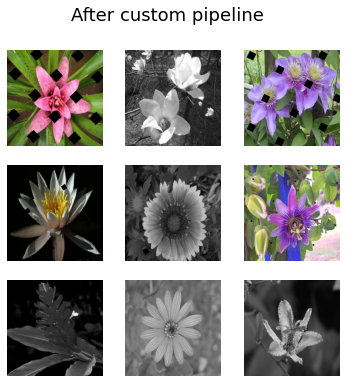Looks great! You can use `RandomAugmentationPipeline` however you want.

## Training a CNN

As a final exercise, let's take some of these layers for a spin. In this section, we will use `CutMix`, `MixUp`, and `RandAugment` to train a state of the art `ResNet50` image classifier on the Oxford flowers dataset.

``````def preprocess_for_model(inputs):
images, labels = inputs["images"], inputs["labels"]
images = tf.cast(images, tf.float32)
return images, labels

train_dataset = (
.map(apply_rand_augment, num_parallel_calls=AUTOTUNE)
.map(cut_mix_and_mix_up, num_parallel_calls=AUTOTUNE)
)

visualize_dataset(train_dataset, "CutMix, MixUp and RandAugment")

train_dataset = train_dataset.map(preprocess_for_model, num_parallel_calls=AUTOTUNE)

test_dataset = test_dataset.map(preprocess_for_model, num_parallel_calls=AUTOTUNE)

train_dataset = train_dataset.prefetch(AUTOTUNE)
test_dataset = test_dataset.prefetch(AUTOTUNE)
``````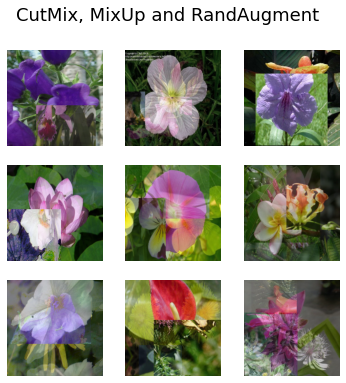Next we should create a the model itself. Notice that we use `label_smoothing=0.1` in the loss function. When using `MixUp`, label smoothing is highly recommended.

``````input_shape = IMAGE_SIZE + (3,)

def get_model():
model = keras_cv.models.ImageClassifier.from_preset(
"efficientnetv2_s", num_classes=num_classes
)
model.compile(
loss=losses.CategoricalCrossentropy(label_smoothing=0.1),
optimizer=optimizers.SGD(momentum=0.9),
metrics=["accuracy"],
)
return model
``````

Finally we train the model:

``````strategy = tf.distribute.MirroredStrategy()
with strategy.scope():
model = get_model()
model.fit(
train_dataset,
epochs=1,
validation_data=test_dataset,
)
``````
``````32/32 [==============================] - 769s 24s/step - loss: 4.7812 - accuracy: 0.0108 - val_loss: 4.6148 - val_accuracy: 0.0241
``````

## Conclusion & next steps

That's all it takes to assemble state of the art image augmentation pipeliens with KerasCV!

• Experiment with custom `RandomAugmentationPipeline` objects.